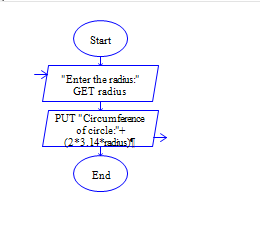# Circumference of Circle in Python

Python program to get radius of circle and find circumference of circle.

Sample Input 1:

4

Sample Output 1:

25.12

#### Flow Chart Design#### Program or Solution

``` r=int(input("Enter the radius:")) area=2*3.14*r print("circumference: {}".format(area)) ```

#### Program Explanation

get the radius of a circle using input() method calculate area = 2 * pi * r